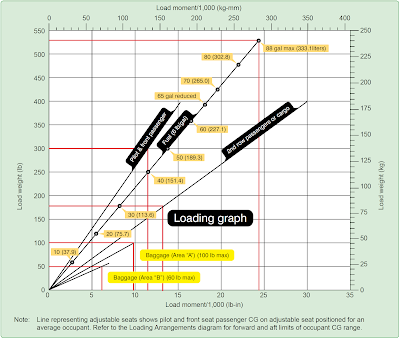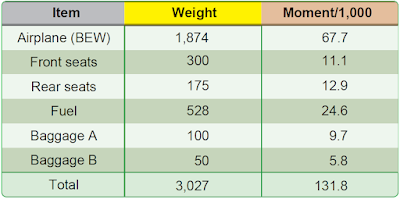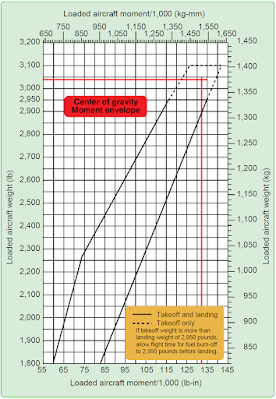# Single-Engine Aircraft Weight and Balance Computations (Part 2)

The charts and graphs found in the POH/AFM can help simplify and expedite the preflight weight and balance computation process. Some use a loading graph and moment indexes rather than the arms and moments. These charts eliminate the need for calculating moments and make computations quicker and easier. [Figure 1]Figure 1. Typical loading graph

### Moment Indexes

Moments determined by multiplying the weight of each component by its arm result in large numbers that are awkward to calculate and can become a source of mathematical error. To eliminate these large numbers, moment indexes are sometimes used. The moment is divided by a reduction factor, such as 100 or 1,000, to get the moment index. The loading graph provides the moment index for each component to avoid mathematical calculations. The CG envelope uses moment indexes rather than arms and moments.

The CG limits envelope is the enclosed area on a graph of the airplane loaded weight and the CG location. If lines drawn from the weight and CG cross within this envelope, the airplane is properly loaded.

Figure 1 is a typical loading graph taken from the POH of a modern four-place airplane. It is a graph of load weight and load moment indexes. Diagonal lines for each item relate the weight to the moment index without having to use mathematical calculations.Figure 2. Loading schedule chartFigure 3. CG moment envelope